Open in App
Not now

## Related Articles

• CBSE Class 9 Syllabus (All Subjects)
• CBSE Class 9 Maths Notes
• What is Vaporization?
• Volume of Cylinder

# Volume of Cylinder

• Last Updated : 19 Mar, 2023

A cylinder is a three-dimensional solid figure with two identical bases connected by a curving surface. It can be visualized as a stack of circular discs which are placed on top of each other. For any cylinder, the perpendicular distance between the bases(both bottom and top) is called the height of a cylinder, and the radius of any of its bases is the radius of the cylinder.

## What is the Volume of the Cylinder?

The volume of a cylinder is the total capacity of any cylinder, i.e. the total amount of liquid any cylinder can hold. It is generally measured in liters but as liters and cubic units are two units that can be converted into each other, the volume can also be measured in m3, cm3, etc, or converted in m3 from liters and vice versa. It is calculated by multiplying the area of the base of the cylinder by its height.

## Volume of a Cylinder Formula

The volume of a cylinder is also equal to the amount of space taken by it in a three-dimensional space. In other words, it determines the space or region enclosed by the cylinder is its volume, and the unit of volume is cubic unit i.e., the number of unit cubes (cubes of unit length) that may fit into an object.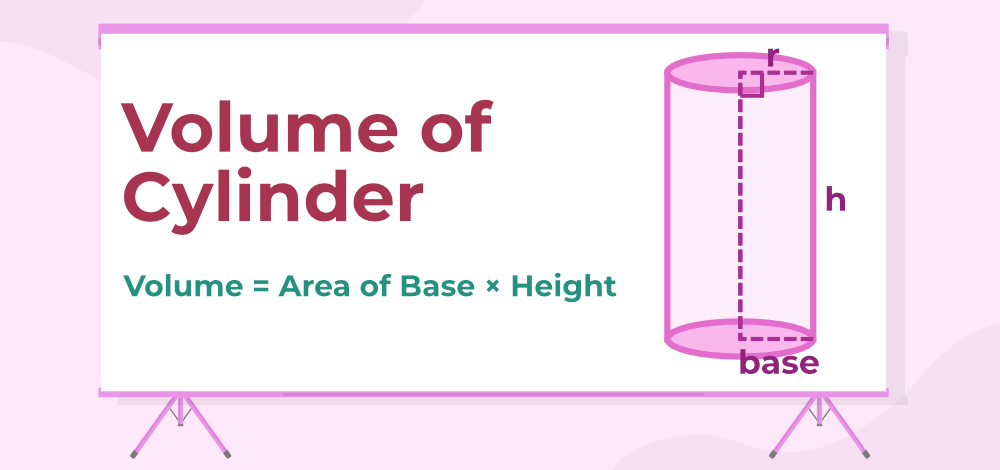## Volume of a Right Circular Cylinder

In the Right Circular Cylinder angle between the plane of the base or top and the curved surface is a right angle. For a right circular cylinder, the base is a circle with radius r, thus its area is πr2, and the height of the cylinder is h then the volume of the cylinder is: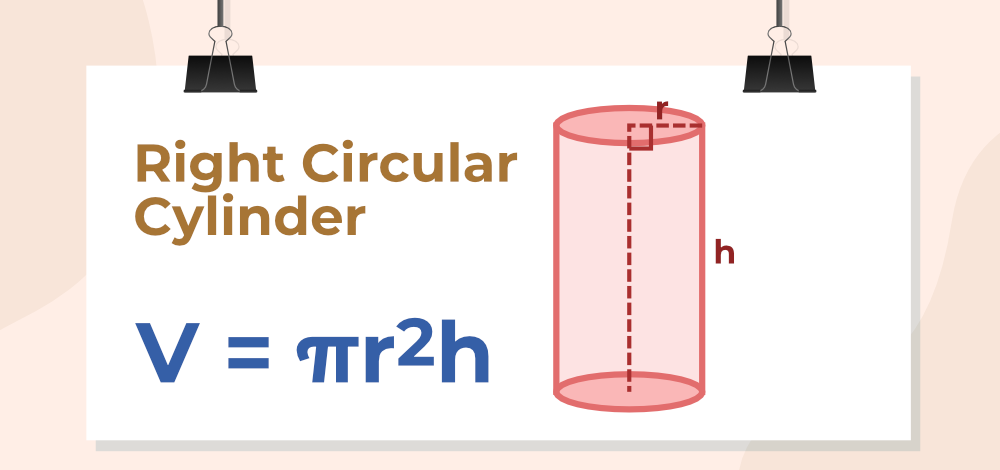## Volume of a Hollow Cylinder

A hollow cylinder is a cylinder that is hollow from the inside thus, its bases form a ring with two radii inner radius and an outer radius. Suppose a hollow cylinder is taken with its inner radius as r and outer radius as R and the height of the cylinder is h then,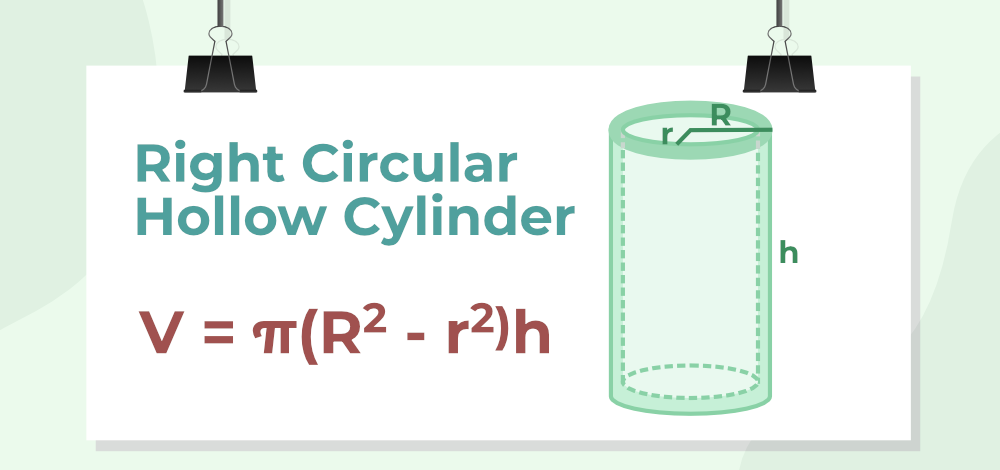## Volume of an Oblique Cylinder

In an Oblique Cylinder angle between the plane of the base or top and the curved surface is not a right angle, but volume is given by the same formula as a product of the area of the base and the height of the cylinder.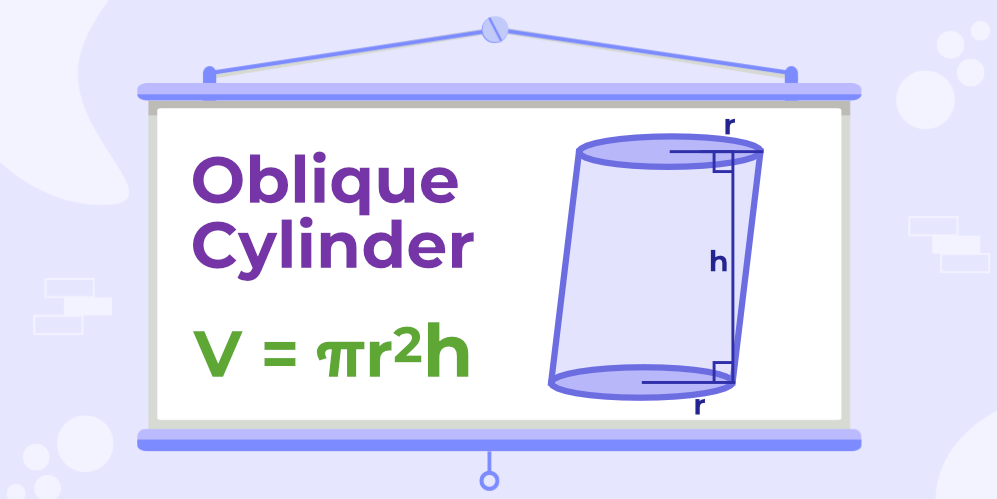## Volume of an Elliptic Cylinder

A cylinder; with an ellipse as its base instead of a circle, is known as Elliptic Cylinder. Let both radii of the base which is an ellipse be a and b and the height of the cylinder be h, then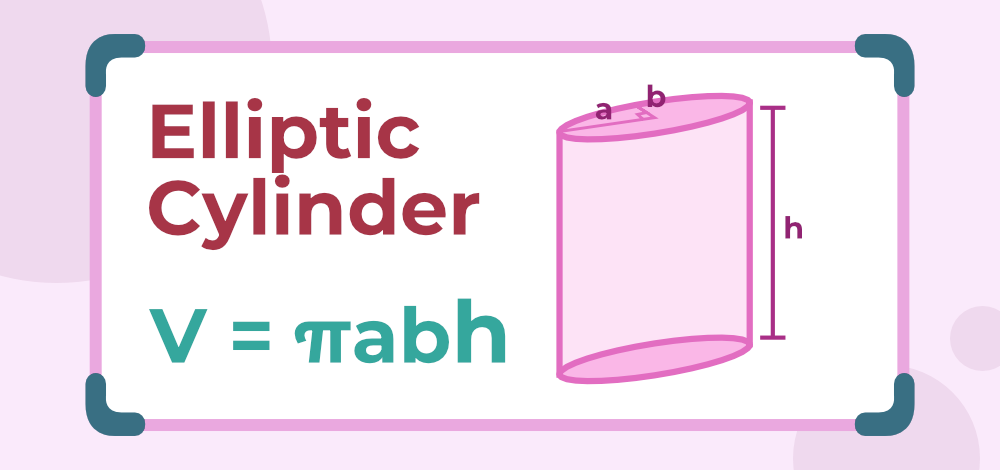## How To Find the Volume of a Cylinder?

Let’s take an example to understand how we can calculate the volume of a cylinder.

Example: Calculate the volume of a cylinder of radius 4 m and height 5 m.

Step 1: Note the radius and height of cylinder. In this example, the radius of the cylinder is 4 m and height is 5 m.

Step 2: We know that the volume of a cylinder is equal to πr2h. Substitute the given value of radius and height in the formula.

Step 3: So, the volume of cylinder is calculated as, 3.14 × (4)2 × 5 = 251.20 cu. m.

## Volume of a Cylinder in Liters

Generally, the volume of a cylinder is calculated in cubic meters or cubic centimeters but we can change them in liters by using the conversion discussed below i.e.,

1 cm3 = 1 ml,

1000 cm3 = 1 litre,

1 m3 = 1000000  cm3 = 1000 litres

Example: If a cylinder has a volume of 32 m3 it can be written as 32×1000 liters = 32000 liters

## Surface Area of a Cylinder

Surface Area of any figure is defined as the area required to totally cover the surface of any object. So, the surface area of a cylinder can be defined as the area required to cover the surface of the cylinder.

### CSA of Cylinder

Curved Surface Area or CSA is the area of the surface that is not flat but rounded. So, the CSA of the cylinder is all the surface except the base and top. If r is the radius of the base and h is the height of the cylinder, then CSA is given as follows:

CSA of Cylinder = 2πrh

### TSA of Cylinder

Total Surface Area or TSA is the area of the completer surface of the cylinder. So, TSA is the sum of the CSA and the area of the top as well as the base of the cylinder. So, if the base radius of the cylinder is r and the height of the cylinder is h then TSA is given by,

TSA of cylinder = 2πr(r+h)

## Solved Problems on the Volume of the Cylinder

Problem 1: Calculate the volume of a cylinder of radius 3 m and a height of 4 m. (take π = 3.14)

Solution:

We have, r = 3 and h = 4

Using the formula we have,

V = πr2h

⇒ V = 3.14 × (3)2 × 4

⇒ V = 113.04 m3

Problem 2: Calculate the volume of a cylinder of radius 4 m and a height of 7 m.

Solution:

We have, r = 4 and h = 7

Using the formula we have,

V = πr2h

⇒ V = 3.14 × (4)2 × 7

⇒ V = 351.68 m3

Problem 3: Calculate the radius of a cylinder if its volume is 300 m3 and height is 7 m.

Solution:

We have, V = 300 and h = 7

Using the formula we have,

V = πr2h

⇒ r2 = V/πh

⇒  r2 = 300/(3.14 × 7)

⇒  r = 3.68 m

Problem 4: Calculate the radius of a cylinder if its volume is 450 m3 and its height is 9 m.

Solution:

We have, V = 450 and h = 9

Using the formula we have,

V = πr2h

⇒ r2 = V/πh

⇒ r2 = 450/(3.14 × 9)

⇒ r = 12.52 m

Problem 5: Calculate the height of a cylinder if its volume is 570 m3 and its radius is 4 m.

Solution:

We have, V = 570 and r = 4

Using the formula we have,

V = πr2h

⇒ h = V/πr2

⇒ h = 570/(3.14 × 4 × 4)

⇒ h = 11.34 m

Problem 6: Calculate the height of a cylinder if its volume is 341 m3 and its radius is 6 m.

Solution:

We have,

V = 341 m3

r = 6 m

Using the formula we have,

V = πr2h

⇒ h = V/πr2

⇒ h = 341/(3.14 × 6 × 6)

⇒ h = 3.01 m

## FAQs on Volume of Cylinder

### Question 1: What is meant by the volume of a cylinder?

The volume of a cylinder is defined as the capacity of the cylinder, i.e, the amount of substance a cylinder can hold. It can also be defined as the total material required for making a cylinder.

Volume of the cylinder (V) is given by,

V = (Area of circular base) × (Height)

V = πr2h

where,

h is height of cylinder

### Question 2: By what factor is the volume of the cylinder changed if its radius is doubled?

We already know that volume of cylinder is directly proportional to the square of its radius.(volume of cylinder ∝ Radius2)
So if the radius is doubled, (i.e., r = 2r), we get
V = πr2
⇒ V = π(2r)2
⇒ V = 4πr2h.
Hence, the volume of cylinder becomes four times its initial value, when its radius is doubled.

### Question 3: By what factor is the volume of the cylinder changed if its radius is halved?

We already know that volume of cylinder is directly proportional to the square of its radius.(volume of cylinder ∝ Radius2)
So if the radius is halved, (i.e., r = r/2), we get
V = π(r/2)2
⇒ V = (πr2h)/4
Thus, the volume of cylinder becomes one-fourth its initial value, when its radius is halved.

### Question 4: What is the unit for the volume of a cylinder?

Volume of a cylinder is normally measured in cubic units, i.e. cubic centimeters (cm3), cubic meters (m3), cubic feet (ft3) and so on for mathematical purposes. In general usage, it is measured in Liters(l), milliliters(ml), etc.